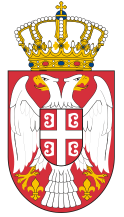Dokument se učitava

# Soft ComputingCourse code: 130317 | 6 ECTS credits

Basic information
Level of Studies: Undergraduate applied studies
Year of Study: 2
Semester: 4
Requirements:
Goal: Introducing students to basic concepts of soft computing, and, in parts, machine learning.
Outcome: Students will be trained to use soft computing concepts and certain machine learning algorithms when designing software products and solutions that are partly based on these concepts.
Contents of the course
Theoretical instruction:
1. Basic concepts of mathematical logic, artificial intelligence, and soft computing.
2. Fuzzy logic. Fuzzy relations, logic and conclusion methods.
3. Artificial neural networks. Neuron, activation functions, network architectures.
4. Model training, cross-validation, over-fitting problem.
5. Deep learning.
6. Support Vector Machines.
7. Anomaly theory and anomaly detection methods.
8. Evolutionary computing.
9. Genetic algorithms.
10. Nature-inspired meta-heuristics and optimization problems.
11. Artificial immune systems.
12. Probabilistic computing. Theory of probability, game theory and decision theory. Bayesian Networks.
13. The chaos theory. Deterministic chaos, synchronization of chaotic systems, classic chaotic communication models.
14. Rough sets.
15. Soft computing applications.
Practical instruction (Problem solving sessions/Lab work/Practical training):
1. Practicing presented concepts, methods and techniques through examples.
Textbooks and References
1. M. Milosavljević, "Veštačka inteligencija", Univerzitet Singidunum, 2015.
2. V. Miškovic, "Sistemi za podršku odlučivanju", Univerzitet Singidunum, 2013.
3. E. Volna, "Introduction to Soft Computing", Bookbon & Eva Volna, 2013.
4. D. K. Pratihar, "Soft computing: fundamentals and applications", Alpha Science International, Ltd., 2013.
5. G. Tettamanzi, M. Tomassini, "Soft computing: integrating evolutionary, neural, and fuzzy systems", Springer Science & Business Media, 2013.
6. V. Chandola, A. Banerjee, V. Kumar, "Anomaly detection: A survey", ACM computing surveys, 2009.
Number of active classes (weekly)
Lectures: 3
Practical classes: 2
Other types of classes: 0
Grading (maximum number of points: 100)
Pre-exam obligations
Points
activities during lectures
0
activities on practial excersises
20
seminary work
0
colloquium
40
Final exam
Points
Written exam
40
Oral exam
0